Next: Mean-field calculations for other Up: Time-reversal violating Schiff moment Previous: Introduction

# Enhancement of Schiff Moments in Octupole-Deformed Nuclei - Previous Work

In analogy with dipole moments in atoms, static Schiff moments in nuclei can exist only if T is broken. Because T-violating forces are much weaker than the strong interaction, the Schiff moment can be accurately evaluated through first-order perturbation theory as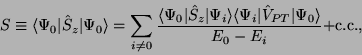(2)

where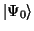is the member of the ground-state multiplet with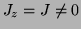, the sum is over excited states, and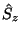is the operator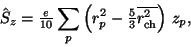(3)

with the sum here over protons. The operator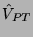is the T- (and parity-) violating nucleon-nucleon interaction mediated by the pion [Haxton and Henley(1983),Herczeg(1988a)] (shown to be more important than other mesons in Ref. [Towner and Hayes(1994)]):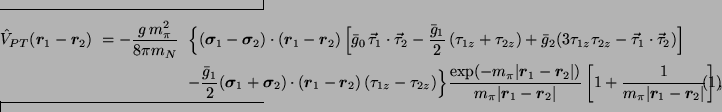where arrows denote isovector operators,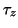is +1 for neutrons,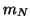is the nucleon mass, and we are using the convention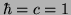. The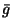's are the unknown isoscalar, isovector, and isotensor T-violating pion-nucleon couplings, and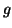is the usual strong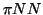coupling.

In a nucleus such as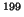Hg, with no intrinsic octupole deformation, many intermediate states contribute to the sum in Eq. (2). By contrast, the asymmetric shape of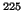Ra implies the existence of a very low-energy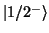state, in this case 55 keV above the ground state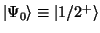, that dominates the sum because of the corresponding small denominator. To very good approximation, then,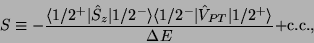(4)

where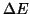= 55 keV. The small denominator is part of the reason for the enhancement of the Schiff moment. The other part is the matrix element of the Schiff operator in Eq. (5). In the limit that the deformation is rigid, the ground state and first excited state in octupole-deformed nuclei are partners in a parity doublet, i.e., projections onto good parity and angular momentum of the same intrinsic state" that represents the wave function of the nucleus in its own body-fixed frame. The matrix elements in Eq. (5) are then proportional (again, in the limit of rigid deformation) to intrinsic-state expectation values, so that [Spevak et al.(1997)Spevak, Auerbach, and Flambaum]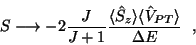(5)

where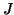is the ground-state angular momentum, equal to 1/2 forRa, and the brackets indicate expectation values in the intrinsic state. The intrinsic-state expectation value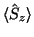is generated by the collective quadrupole and octupole deformation of the entire nucleus; it is much larger than a typical matrix element in a spherical or symmetrically deformed nucleus. Together with the small energy denominator, this large matrix element is responsible for the enhancement of laboratory-frame Schiff moments in nuclei such asRa.

The amount of the enhancement is not easy to calculate accurately, however. The reason is that the matrix element of the two-body spin-dependent operatorin Eq. (5) depends sensitively on the behavior of a few valence particles, which carry most of the spin. In the approximation that particles (or quasiparticles) move in independent orbits generated by a mean field, the potential can be written as an effective density-dependent one-body operator that we will denote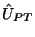, defined implicitly by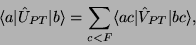(6)

where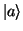,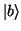, and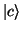are eigenstates of the mean field and the matrix elements ofare antisymmetrized. With the further approximation that the mass of the pion is very large,can be written as a local operator, in a form we display in the Section IV. Evaluating its matrix element is tricky.

The authors of Refs. [Spevak et al.(1997)Spevak, Auerbach, and Flambaum,Auerbach et al.(1996)Auerbach, Flambaum, and Spevak] used a version of the particle-rotor model [Leander and Sheline(1984)] to represent the odd-nucleus. In this model, all but one of the nucleons are treated as a rigid core, and the last valence nucleon occupies a deformed single-particle orbit, obtained by solving a Schrödinger equation for a Nilsson or deformed Wood-Saxon potential. The model implies that the core carries no intrinsic spin whatever, that the neutron and proton densities are proportional, and that the exchange terms on the right-hand side of Eq. (7) are negligible. Under these assumptions,, which now acts only on the single valence nucleon, reduces to [Sushkov et al.(1984)Sushkov, Flambaum, and Khriplovich]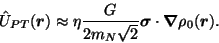(7)

where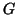is the Fermi constant, inserted to follow convention, and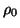is the total nuclear mass density. The dimensionless parameter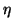is then a function of the couplings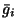and the isospin of the nucleus.

Ref. [Engel et al.(1999)Engel, Friar, and Hayes] confirmed the collectivity of the intrinsic Schiff moments obtained in Refs. [Spevak et al.(1997)Spevak, Auerbach, and Flambaum,Auerbach et al.(1996)Auerbach, Flambaum, and Spevak], but questioned the accuracy of some of the assumptions used to evaluate the matrix element of, suggesting that either core-spin polarization or self-consistency in the nuclear wave function might reduce laboratory Schiff moments. The zero-range approximation and the neglect of exchange inare also open to question. As a result, it is not clear whether the Schiff moment ofRa is 1000 times that ofHg or 100 times, or even less. In what follows, we provide a (tentative) answer by moving beyond the particle-rotor model. Our calculation is not the final word on Schiff moments in octupole-deformed nuclei -- we only do mean-field theory, neglecting in particular to project onto states with good parity, and do not fully account for the pion's nonzero range -- but is a major step forward.Next: Mean-field calculations for other Up: Time-reversal violating Schiff moment Previous: Introduction
Jacek Dobaczewski 2003-04-24